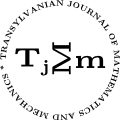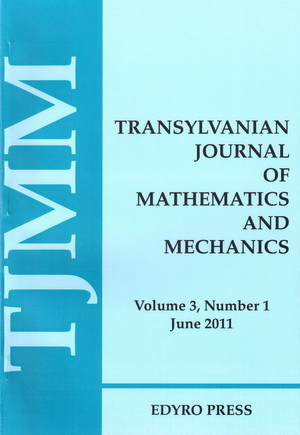ISSN: 2067-239X
ISSN(on-line): 2067-239X

Indexed in:
Mathematical Reviews
Zentralblatt MATH
EBSCO

Front cover# Volume 14 (2022), Number 1

## Fixed Point Theorem for a Meir-Keeler Type Mapping in a Metric Space With a Transitive Relation

### Author(s): KOJI AOYAMA and MASASHI TOYODA

Abstract: The aim of this paper is to provide characterizations of a Meir-Keeler type mapping and a fixed point theorem for the mapping in a metric space endowed with a transitive relation.

## A New Way of Finding Trapezium Inequality Involving Harmonic Convex Functions Through Generalized Fractional Integrals

Abstract: The main objective of this paper is to obtain some new trapezium type inequalities essentially involving the class of harmonic convex functions and generalized fractional integrals.

## On Some Refinements of Jensen and Related Inequalities With Applications

### Author(s): SADIA CHANAN and ASIF R. KHAN

Abstract: The aim of this article is to give the cyclic refinement of the Jensen’s inequality, its variant and extension by considering real weights.Applications to Ky Fan inequality and cyclic mixed symmetric means are also given.

## On the Practical Stabilization of Infinite-Dimensional Perturbed Systems

### Author(s): HANEN DAMAK and MOHAMED ALI HAMMAMI

Abstract: In this paper, we investigate the notion of practical feedback stabilization of a class of non-autonomous infinite-dimensional systems. Assuming appropriate conditions on the perturbation term, it is shown that if every frozen-time control system is stabilizable then the corresponding non-autonomous infinite-dimensional control system is practically stabilizable. Sufficient conditions for the practical feedback stabilizability on a separable Hilbert space are given. This approach is based on the freezing method. Some examples are considered to illustrate the result obtained.

## The Vigenère Cipher

### Author(s): ANA-MARIA DOBRIȚOIU

Abstract: This paper contains an overview to a well-known encryption method, Vigenère’s cipher, which although easy to understand and implement, seems impossible for beginners to break, which is why it has been described as ”le chiffre indéchiffrable”.

## On the Addition of a Dirac Mass to a $q$-Laguerre-Hahn Form

### Author(s): S. JBELI and L. KHÉRIJI

Abstract: Our goal is to study the addition of a Dirac mass to a $Hq$-Laguerre-Hahn form where $Hq$ be the $q$-derivative operator. The $Hq$-Laguerre-Hahn character and the class of the obtained form is discussed into detail. An example in connection with the first order associated of a $Hq$-classical form is highlighted.

## A New Efficient Strategy for Solving the System of Cauchy Integral Equations via Two Projection Methods

### Author(s): ABDELAZIZ MENNOUNI

Abstract: It is interesting to solve a system of singular integral equations with bounded non-compact operators by projection methods in Hilbert space. The theory of projection methods for solving a system of Cauchy integral equations in $L2([ 0,1 ],ℂ )×L2([ 0,1 ] ,ℂ)$ is developed and extended in this work. We look at two situations: Galerkin approximations and Kulkarni approximations. This is accomplished through the use of a sequence of orthogonal finite rank projections. A new efficient technique is used to obtain an equivalent system of two separable equations. The existence of the approach solution, as well as the error analysis, are established. A numerical example illustrates theoretical results.

## Corruption in the EU-27. Time Analysis of the Corruption Perception Index

### Author(s): STOICUTA NADIA ELENA and STOICUTA OLIMPIU

Abstract: One of the most targeted issues in the world today is the reduction of corruption. As efforts to combat this deeply damaging phenomenon stagnate around the world, human rights and democracy are under attack. According to studies, most countries in the world have not seen significant declines in corruption in the last decade. The global pandemic with COVID-19 virus has also been used in many countries as an excuse to avoid both medical and financial controls. Based on these considerations, this article proposes an econometric analysis of the evolution of the corruption perception index over time for five EU-27 Member States, namely Denmark, Germany, Poland, Romania and Bulgary. Based on the econometric models analyzed for each state, short-term forecasts of 3 years will be made.

## Nonholonomic Nonlinear Systems: A General Energy Equation

### Author(s): FEDERICO TALAMUCCI

Abstract: Nonholonomic systems with nonlinear restrictions with respect to the velocities are considered. The mathematical problem is formulated by means of the Voronec equations extended to the nonlinear case. The double formulation both by means of the vector of accelerations and the Lagrangian function turns out to be convenient depending on the aspects and the properties to be pointed out. The main point of the paper is the balance of the mechanical energy induced by the equations of motion; the conservation of the energy on the basis of the tipology of the constraint equations is discussed. The special form of the energy equation allows to identify the categories of nonlinear constraints which entail the conservation of energy.

## Notes on the n-th Power of Generalized $(S,T)$-Pell and $(S,T)$-Pell Lucas Matrix Sequences
Abstract: In this study, we define two matrix sequences special $2×2$ matrices are defined with elements that are generalizations of Pell and Pell-Lucas sequences. Some formulas for the nth powers of these special matrix sequences are established by determinant and trace of these matrices. By these formulas, some properties for these generalized number sequences are demonsrated. The results are also usable for the classic Pell and Pell Lucas numbers, if we substitute for $s =t=1$.